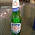## Wednesday, November 13, 2013

### HP Prime Programming Tutorial #4: CHOOSE and CASE, Tip about INPUT

Welcome to Part 4 of our programming series for the Prime. Today's session will cover CHOOSE and CASE.

First a tip from Han of the MoHPC Forum, which is found at http://www.hpmuseum.org/cgi-sys/cgiwrap/hpmuseum/forum.cgi#255084. Thank you Han for allowing me to share this.

Use the IF THEN ELSE structure with INPUT to execute a set of default instructions if the user presses cancel. INPUT returns a value of 0 if ESC or cancel is pressed, and 1 if a value is entered.

IF INPUT(...) THEN
commands if values are entered
ELSE
commands if Cancel is pressed
END;

Default values can be assigned to values as an optional fifth argument for INPUT.

INPUT(var, "Title", "Prompt", "Help", default value)

The type of variable maybe set to other than real numbers. Just remember to store such type before the INPUT command. For example, if you want var to be a string, store an empty string:

var:=" ";

Again, major thanks to Han.
CHOOSE and CASE

CHOOSE: Creates a pop up choose box, similar to what you see when you click on a soft menu. There are two syntaxes for CHOOSE:

Simple Syntax (up to 14 options):
CHOOSE(var, "title string", "item 1", "item 2", ... , "item n");

List syntax (infinite amount of items):
CHOOSE(var, "title string", {"item 1", "item 2"});

Choosing item 1 assigns the value of 1 to var, choosing item 2 assigns the value of 2 to var.

Access: Cmds, 6. I/O, 1. CHOOSE

CASE: Allows for different test cases for one variable. Also includes a default scenario (optional).

CASE
IF test 1 THEN do if true END;
IF test 2 THEN do if true END;
...
DEFAULT commands END;

Access: Cmds, 2. Branch, 3. CASE

Let's look at two programs to demonstrate both CHOOSE and CASE.
TERMVEL - Terminal Velocity of an Object

EXPORT TERMVEL()
BEGIN

LOCAL L0:={9.80665,32.174},
L1:={1.225,.0765},
L2:={.47,1.05,1.15,.04},C,K,M,A,T;

CHOOSE(C,"Units","SI","English");

CHOOSE(K,"Type of Object","Sphere","Cube",
"Cylinder","Tear-Shaped");

INPUT({M,A},"Object",
{"M=","A="},{"Mass","Surface Area"});

T:=√((2*M*L0(C))/(L1(C)*A*L2(K)));

MSGBOX("Terminal Velocity="+T);
RETURN T;
END;

Examples:

Sphere, SI Units, M = .05 kg, A = .0028 m^2
Terminal Velocity: T = 24.6640475387 m/s

Cube, US Units, M = 1.2 lb, A = .3403 ft^2
Terminal Velocity: T = 53.149821209 ft/s

AREAC - Area of Circles, Rings, and Sectors

EXPORT AREAC()
BEGIN
LOCAL C,R,S,θ,A;
CHOOSE(C,"Areas","1. Circle","2. Ring","3. Sector");

INPUT(R, "Input Radius", "R =");

CASE
IF C==1 THEN A:=π*R^2; END;

IF C==2 THEN
A:=π*(R^2-S^2);
END;

IF C==3
INPUT(θ, "Angle", "θ=");
\\ Assume you are in the correct angle mode
IF HAngle==1 THEN
\\ Test Angle Mode
θ:=θ*π/180;
END;
A:=θ*R^2/2;
END;

END;

MSGBOX("Area is "+A);
RETURN A;
END;

Examples

R = 2.5, r = 1.5, θ = π/4 radians or 45°

Circle: 19.6349540849
Ring: 12.5663706144
Sector: 2.45436926062

That is how, in general CHOOSE and CASE work. I thank you as always. It is so good to finally be rid of a cold and firing on all cylinders again.

Eddie

This blog is property of Edward Shore. 2013

#### 1 comment:

1.Very useful, thanks.

Is there any way to return back to the start of the program by capturing the Escape Key? i.e. Can you exit the solver app but return control back to the calling program and let the user exit via an option from a message box?

### Retro Review: Radio Shack EC-2024

Retro Review:  Radio Shack EC-2024 Quick Facts Company:  Radio Shack Years in Production: Around 1994 Original Price: \$24.99 Bat...